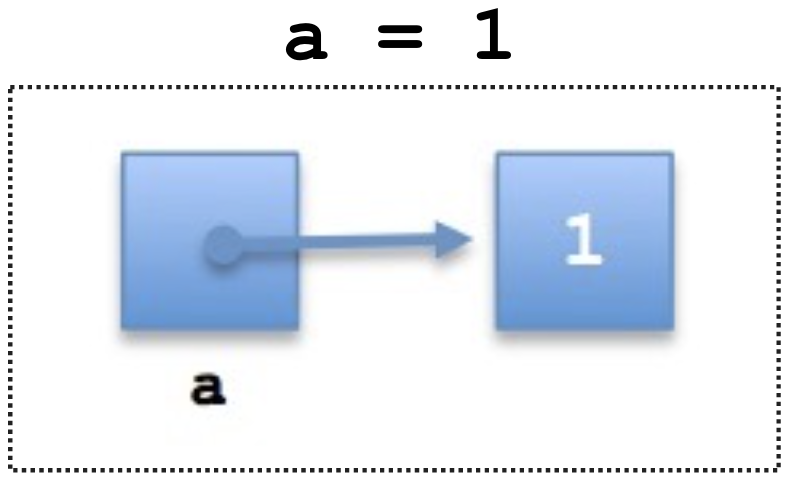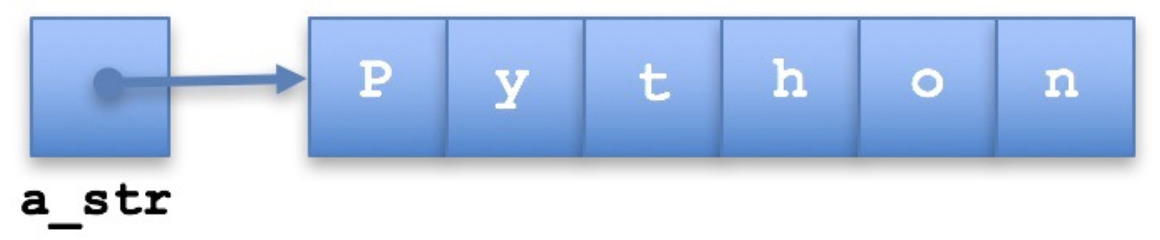ControlNet

## 数据类型和数据结构

Python中的数据类型可以分为两种，

• 原子类型(Atomic)
• 集合类型(Collective)

### 数据结构

• 数据结构是计算机科学理论中的一般术语。
• 数据类型是某个特定的编程语言中的数据结构的实现。

• 原始(Primitive)数据结构(布尔Boolean, 浮点Floating-point, 整数Integer)
• 非原始(Non-primitive)数据结构(数组Array, 列表List)
• 线性结构(栈Stack, 队列Queue)
• 非线性结构(图Graph, 树Tree, 哈希表Hashmap)

## Python的集合数据类型

• 字符串str
• a = "Hello"
• 列表list
• a = [1, 3, 5]
• 元组tuple
• a = (6, 2)
• 集合set
• a = {1, 5, 3}
• 字典dictionary
• a = {"a": "b", 1: 2}

• 一个Python对象(和它的值)

• 指向另一个内存地址的引用### 字符串• 第一个字符: a_str
• 第二个字符: a_str
• 最后一个字符: a_str[len(a_str) - 1] 或者 a_str[-1]

• str.capitalize() 像标题一样每个单词大写第一个字符
• str.count() 返回这个子字符串在这个字符串中的出现次数
• str.endswith() 返回这个字符串是否是有这个后缀
• str.find() 查找子字符串的位置
• str.index() 返回所给的子字符串的index
• str.isdigit() 这个字符串是否全是数字
• str.join() 将list的全部元素都合并成一个字符串
• str.lower() 转换成小写
• str.replace() 替换其中的某个子字符串
• str.split() 通过某个分隔符分割成列表
• str.strip() 去除首尾的空格

### 序列

Python中的序列(Sequence)是一个数据的集合。这些数据中的元素都可以读取，而有些序列还可以修改其中的元素。

• 列表(list), 元组(tuple), 集合(set), 字典(dict)

• in表达式:
• 检查这个元素是不是在这个序列中
• 拼接
• 合并两个序列，一般用”+”运算符
• 通过下标取值c
• 通过一个给定的下标，取其中的一个值，在python中用中括号[]来操作
• 切片
• 通过两个下标，开始的位置和结束的位置，取一个子序列
• 一些基础分析
• min, max, seq.index, seq.count等等

in表达式

### 列表

• 可以保存多个任意类型的数据
• 可变的(Mutable)，而且是有顺序的

• 创建空列表: a_list = [], a_list = list()
• 创建一个带有元素的列表: a_list = [1, "two", 3.0, '4']
• 创建一个带有相同元素的列表: a_list =  * 6

index     
value “Apple” “Mango” “Strawberry” “Banana” “Guava”

• 添加到末端: a_list.append(new_item)
• 添加到指定位置: a_list.insert(index, new_item)

• 移除末尾或者指定位置，并返回这个元素: a_list.pop()a_list.pop(index)
• 移除指定值的元素: a_list.remove(item)

• 按照值的顺序进行排列，默认升序: a_list.sort()
• 直接将元素倒序: a_list.reverse()

### 元组

• 空元组: a_tuple = ()
• 有一些值的元组: a_tuple = (0, "a")
• 列表转换到元组: a_tuple = tuple([0, "a"])

• 使用下标: a_tuple
• 解构赋值给新变量: x, y = a_tuple

• 列表主要代表一个有顺序的序列，而元组是表示一个结构
• 列表是可变的，元组是不可变的
• 元组比列表更节省内存